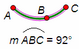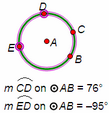# Arc AngleSelection prerequisites: One of the following:

One or more arcs

A circle and two points on the circle

A circle and three points on the circle

This Measure menu command measures the angle of each selected arc using the angle units chosen on the Units panel of the Preferences dialog box.

 If one or more arcs are selected, this command measures the angle of each selected arc. If the angle units in Preferences are set to degrees or directed degrees, the value ranges from 0° to 360°. If the angle units are set to radians, possible values range from 0 radians to 2π radians. Arc angle (sometimes also called arc measure) refers to the central angle — that is, the angle formed by the radii connecting the circle’s center to the endpoints of the arc, as shown here.If a circle and two points are selected, this command measures the angle of the minor arc on the circle defined by the two selected endpoints. If the angle units in Preferences are set to degrees, possible values range from 0° to 180°. If the angle units in Preferences are set to directed degrees, values range from –180° to 180°; clockwise arcs result in negative values, and counter-clockwise arcs result in positive values. If the angle units are set to radians, possible values range from –π radians to π radians.If a circle and three points are selected, this command measures the angle of the minor or major arc that starts at the first selected point, passes through the second point, and ends at the third point. If the angle units in Preferences are set to degrees, possible values range from 0° to 360°. If the angle units in Preferences are set to directed degrees, possible values range from –360° to 360°; clockwise arcs result in negative values, and counter-clockwise arcs result in positive values. If the angle units are set to radians, possible values range from –2π radians to 2π radians.